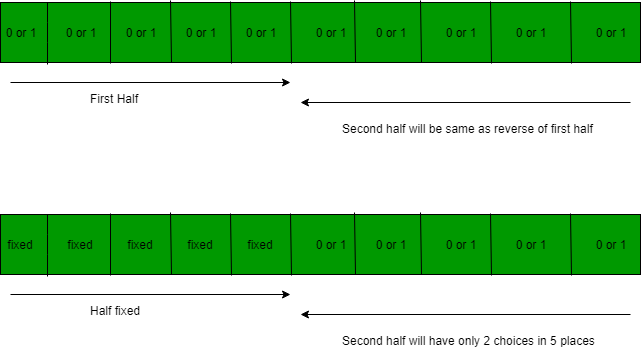Open In App

# UGC-NET | UGC NET CS 2016 Aug – II | Question 4

What is the probability that a randomly selected bit string of length 10 is a palindrome?
(A) 1/64
(B) 1/32
(C) 1/8
(D) 1/4

Explanation: In the given question we have a palindrome: in even length palindrome half length is fixed and rest is repeated. So, in 10 bit palindrome, we have 5 position to be filled with 2 choices each-i.e. 25 choices for first half and 25 choices for second half.

```Probability = favorable outcome / total outcome
=  25/ 210
=  1 / 25
=  1 / 32.```

So, option (B) is correct.

Quiz of this Question